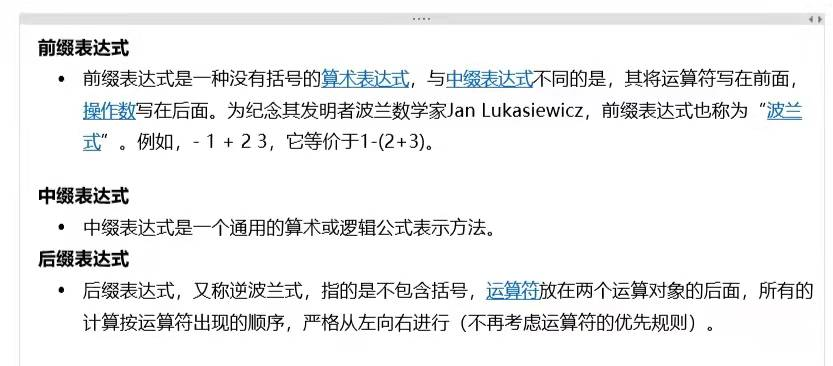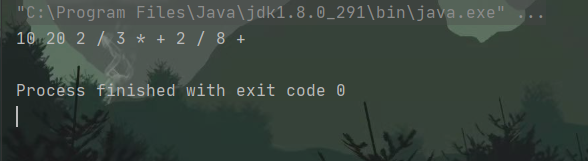• 试题 I:后缀表达式（套题）
2021-03-06 22:45:36

试题 I:后缀表达式

如题~

【问题描述】
给定 N个加号、M个减号以及 N+M+1个整数 A1,A2,···,AN+M+1，小
明想知道在所有由这N个加号、M个减号以及N+M+1个整数凑出的合法的
后缀表达式中，结果最大的是哪一个？
请你输出这个最大的结果。
例如使用123±，则“23+1-”这个后缀表达式结果是4，是最大的。
【输入格式】
第一行包含两个整数N和 M。
第二行包含N+M+1个整数A1,A2,···,AN+M+1。
【输出格式】
输出一个整数，代表答案。
【样例输入】
11
123
【样例输出】
4
【评测用例规模与约定】
对于所有评测用例，0≤N,M≤100000−10e9 ≤Ai≤10e9

首先这个题你需要知道后缀表达式是什么。

。开始我不知道，磨了好久，看的别家大佬的，百度的，我才懂。

下面讲解开始：

后缀表达式不再引用括号，也就是说，你想在哪里用括号就在哪里用括号就行。

比如给你5个数，一个 + 和三个 - 号。

如果，五个数 1 2 3 4 5 全是正数的话。答案自然就是 5 + 4 - 3 - 2 - 1

但是如果五个数是 1 -2 -3 -4 -5，这又怎么算呢。可以这样算

1-（-5）-（-4）-（-3）+（-2）这是正常思路。

但也可以这样做，在后缀表达式中。
1-（-5）-（（-4）+（-3））-（-2），这个答案比上面那个数还要大


多找一些数字，可以总结规律

如果全是加号，答案就是所有数字直接相加。

如果存在减号：
如果全是正数，那么至少有一个被减去，所以把最小的那个减去即可。
如果有正有负，可以利用上面的例子，总是可以把所有数的绝对值加起来。
如果全是负数，除了绝对值最小的负数，给他留着。其他的全部可以变为正数。

代码如下：

#include<bits/stdc++.h>
using namespace std;
long long int a, sum = 0, minx = 1000000001;
int n, m, fsign = 0;
int main()
{
scanf("%d%d", &n, &m);//n m 的值
for (int i = 0; i < n + m + 1; i++)
{
scanf("%lld", &a[i]);
if (a[i] < 0)   fsign++;

sum += a[i];
minx = min(minx, a[i]);
}
if (m == 0)     printf("%lld\n", sum);//没有负号存在时，sum就是所有数的和
else//负号存在
{
if(fsign == 0)//负数为零情况下，就是所有数之和，再-最小值两遍就行。（详见开头讲解）
{
printf("%lld\n", sum - minx - minx);
}
else
{
if (fsign == n + m + 1)  //全是负数的情况，自然有办法给他弄成整数
{
sum = 0; minx = 1000000001;
for (int i = 0; i < n + m + 1; ++i)
{
sum += abs(a[i]);//都是绝对值之和
minx = min(minx, abs(a[i]));//把绝对值最小挑出来减去
}
printf("%lld\n", sum - minx - minx);
}
else
{
sum = 0;
for (int i = 0; i < n + m + 1; ++i)
{
sum += abs(a[i]);
}
printf("%lld\n", sum);
}
}
}
return 0;
}


应该是对的。。算法 c++
更多相关内容
• 本实验取材于浙江大学《数据结构》，计算后缀表达式，关键在于理解堆栈的工作顺序 //堆栈的应用案例--表达式求值 //表达式求值的基本步数 //1当读入的是一个运算数时，把它被压入栈中； //2当读入的是一个运算符时，...

本实验取材于浙江大学《数据结构》，计算后缀表达式，关键在于理解堆栈的工作顺序

//堆栈的应用案例--表达式求值
//表达式求值的基本步数
//1当读入的是一个运算数时，把它被压入栈中；
//2当读入的是一个运算符时，就从堆栈中弹出适当数量的运算数
//对该运算进行计算，计算结果再压回到栈中。
//处理完整个后缀表达时之后，堆栈顶上的元素就是表达时的结果值


下面利用堆栈的顺序存储进行运算！


#include<stdio.h>
#include<stdlib.h>
#include<ctype.h>
#define MAXOP 100//操作数序列可能的最大长度
#define INFINITY 1e9//代表正无穷
#define ERROR -1
typedef double ElementType;//将堆栈的元素类型具体化
//类型依次对应运算符、运算符、字符串结尾

typedef enum{num,opr,end} Type;
typedef int Position;
typedef struct SNode *PtrToStack;

struct SNode{
ElementType *Data;
Position Top;
int MaxSize;
};
typedef PtrToStack Stack;
Stack CreateStack(int MaxSize);//生成空堆栈，其最大长度为Maxsize
int IsFull(Stack S);//判断堆栈S是否已满
int Push(Stack S,ElementType X);//将元素item压入堆栈
int IsEmpty(Stack S);//判断堆栈S是否为空
ElementType Pop(Stack S);//删除并返回栈顶元素
Stack CreateStack(int MaxSize){
Stack S=(Stack)malloc(sizeof(struct SNode));
S->Data = (ElementType *)malloc(MaxSize*sizeof(ElementType));
S->Top = -1;
S->MaxSize = MaxSize;
return S;
}
int IsFull(Stack S){
return (S->Top==S->MaxSize-1);
}

int Push(Stack PtrS,ElementType X)
{
if(IsFull(PtrS)){
printf("堆栈满");
return ERROR;
}else{
PtrS->Data[++(PtrS->Top)] = X;
return PtrS->Data[PtrS->Top];
}
}
int IsEmpty(Stack S){
return (S->Top==-1);
}
ElementType Pop(Stack PtrS)
{
if(PtrS->Top==-1){
printf("堆栈空");
return ERROR;
}else{
return (PtrS->Data[(PtrS->Top)--]);
}
}
Type Getop(char *Expr,int *start,char *str)
{
//从*start开始读入下一个对象(操作数或运算符)，并
//保存在字符串str中
int i=0;
while((str=Expr[(*start)++])==' ');
while(str[i]!=' ' && str[i]!='\0')
str[++i]=Expr[(*start)++];
if(str[i]!='\0')//如果读到输入结尾
(*start)--;//start指向结束符
str[i]='\0';//结束一个对象的获取
if(i==0) return end;//读到了结束
else if(isdigit(str) || isdigit(str))
return num;//表示此时str中存的是一个数字
else    //表示str不是空串，又不是数字
return opr; //表示此时str中存的是一个运算符

}
ElementType PostfixExp(char *Expr)
{
Stack S;
Type T;
ElementType Op1,Op2;
char str[MAXOP];
int start=0;
//申请一个新堆栈
S=CreateStack(MAXOP);
Op1 = Op2=0;
while((T=Getop(Expr,&start,str))!= end){
//当为读到输入结束时
if(T==num)
Push(S,atof(str));
else{
if(!IsEmpty(S)) Op2 = Pop(S);
else Op2 = INFINITY;
if(!IsEmpty(S)) Op1 = Pop(S);
else Op2 = INFINITY;
switch(str){
case '+':Push(S,Op1+Op2);break;
case '*':Push(S,Op1*Op2);break;
case '-':Push(S,Op1-Op2);break;
case '/':
if(Op2 != 0.0)
Push(S,Op1/Op2);
else{
printf("falut div is zero\n");
Op2 = INFINITY;
}
break;
default:
printf("fault unknown fault%s\n",str);
Op2 = INFINITY;
break;
}
if(Op2>=INFINITY) break;
}
}
if(Op2<INFINITY)
if(!IsEmpty(S))
Op2=Pop(S);
else
Op2 = INFINITY;
free(S);
return Op2;
}
int main()
{
char Expr[MAXOP];
ElementType f;
gets(Expr);
f=PostfixExp(Expr);
if(f<INFINITY)
printf("%.4f\n",f);
else
printf("function is fault\n");
return 0;
}

展开全文• 一、什么是中缀表达式，后缀表达式 二、中缀表达式转后缀表达式 例题： 中缀表达式：(10+20/2*3)/2+8转换为对应的后缀为 后缀表达式：10 20 2 / 3 * + 2 / 8 + 解题步骤： （1）观察两表达式；后缀明显没有...

一、什么是中缀表达式，后缀表达式二、中缀表达式转后缀表达式

例题：

中缀表达式：(10+20/2*3)/2+8                 转换为对应的后缀为

后缀表达式：10 20 2 / 3 * + 2 / 8 +

解题思路：

（1）观察两表达式；后缀明显没有“（）”这两个符号，其次与中缀相比数字的顺组没有变化，运

算符的顺序发生改变。

（2）找对应的数据结构知识，有顺序且对存储的数据类型，没有要求因此可以利用ArrayList进行

数据的存储；同时运算的顺序发生明显改变与其运算符的优先级有关，所以我们可以通过

ArrayStack利用其的进出栈特性，改变其顺序。

实现过程：

（1）创建一个栈和一个线性表

（2）将中缀表达式定义为一个字符串，通过给运算符和（）两端加上空格，在利用split(“ ”)方法

将字符串转换为String[];

（3）遍历String[]，数组元素为数字直接进入线性表；数组元素为运算符和()，先进栈；

a.元素进栈时首先获取栈顶元素优先级，如果该元素的优先级大于等于栈顶元素，栈顶元素弹栈并

存入线性表；

b.如果该元素为“（”直接进栈；

c.如果栈内为空或者栈顶元素为“（”则直接进栈；

d.如果元素为“）”栈中元素弹栈并存入线性表直到栈顶元素为“（”；

注意：“（）”不进入线性表中；具体进出栈代码中有详细体现及说明；

（4）遍历线性表并进行元素的拼接，输出拼接的结果

代码实现：

package p2.线性结构;
//中缀表达式转后缀表达式
public class InfixToSuffix {
public static void main(String[] args) {
String expression = "(10+20/2*3)/2+8";
expression = infixToSuffix(expression);
System.out.println(expression);
}
static String infixToSuffix(String expression) {
//操作符的栈
ArrayStack<String> opStack = new ArrayStack<>();
//后缀表达式的线性表
ArrayList<String> suffixList = new ArrayList<>();

//格式化表达式
expression = insertBlanks(expression);
String[] tokens = expression.split(" ");
for (String token : tokens) {
//过滤空串
if (token.length() == 0) {
continue;
}
//判断操作符+ - * /
if (isOperator(token)) {
/*
什么时候操作符进栈？
1.如果栈为空
2.如果栈顶是 (
3.如果栈顶是操作符，且优先级比当前token小

什么时候需要将栈顶操作符出栈？
1.栈顶操作符的优先级 >= 当前token
*/
while (true) {
if (opStack.isEmpty() || opStack.peek().equals("(") || priority(opStack.peek()) < priority(token)) {
opStack.push(token);
break;
}
}
} else if (token.equals("(")) {
opStack.push(token);
} else if (token.equals(")")) {
while (!opStack.peek().equals("(")) {
}
opStack.pop();
} else if (isNumber(token)) {
} else {
throw new IllegalArgumentException("wrong char :" + expression);
}
}
while (!opStack.isEmpty()) {
}
//将数字元素和操作符元素进行拼接
StringBuilder sb = new StringBuilder();
for (int i = 0; i < suffixList.size(); i++) {
sb.append(suffixList.get(i));
sb.append(' ');
}
return sb.toString();
}

private static int priority(String token) {
if (token.equals("+") || token.equals("-")) {
return 0;
}
if (token.equals("*") || token.equals("/")) {
return 1;
}
return -1;
}

private static boolean isNumber(String token) {

}

private static boolean isOperator(String token) {
}

//对原表达式进行格式化处理 给所有的非数字字符两边添加空格
private static String insertBlanks(String expression) {
StringBuilder sb = new StringBuilder();
for (int i = 0; i < expression.length(); i++) {
char c = expression.charAt(i);
if (c == '(' || c == ')' || c == '+' || c == '-' || c == '*' || c == '/') {
sb.append(' ');
sb.append(c);
sb.append(' ');
} else {
sb.append(c);
}
}
return sb.toString();
}
}

输出结果；输出结果与开始的预期结果一致；因此代码成功实现转换；

后缀表达式的计算结合博客：

后缀表达式求值_s逐梦少年的博客-CSDN博客

展开全文数据结构
• 一、中缀表达式转后缀表达式算法思想： （1）从左向右开始扫描中缀表达式； （2）遇到数字时，加入后缀表达式 （3）遇到运算符时：   a.若为 '('，入栈；   b.若为 ')'，则依次把栈中的运算符加入后缀表达式，...

## 一、中缀表达式转后缀表达式算法思想：

（1）从左向右开始扫描中缀表达式。
（2）遇到数字时，加入后缀表达式。
（3）遇到运算符时：
a.若为 '('，入栈；
b.若为 ')'，则依次把栈中的运算符加入后缀表达式，直到出现'('，从栈中删除'('
c.若为除括号外的其他运算符，当其优先级高于除'('外的栈顶运算符时，直接入栈。否则从栈顶开始，依次弹出比当前处理的运算符优先级高和优先级相等的运算符，直到一个比它优先级低的或遇到了一个左括号为止。
（4）当扫描的中缀表达式结束时，栈中的所有运算符依次出栈加入后缀表达式。

## 二、中缀表达式转后缀表达式举例：

待处理序列后缀表达式当前扫描元素动作
a/b+(c*d-e*f)/gaa加入后缀表达式
/b+(c*d-e*f)/ga//入栈
b+(c*d-e*f)/g/abb加入后缀表达式
+(c*d-e*f)/g/ab++优先级低于栈顶的/，弹出/
+(c*d-e*f)/gab/++入栈
(c*d-e*f)/g+ab/((入栈
c*d-e*f)/g+(ab/cc加入后缀表达式
*d-e*f)/g+(ab/c*栈顶为(*入栈
d-e*f)/g+(*ab/cdd加入后缀表达式
-e*f)/g+(*ab/cd--优先级低于栈顶的*，弹出*
-e*f)/g+(ab/cd*-栈顶为(-入栈
e*f)/g+(-ab/cd*ee加入后缀表达式
*f)/g+(-ab/cd*e**优先级高于栈顶的-*入栈
f)/g+(-*ab/cd*eff加入后缀表达式
)/g+(-*ab/cd*ef)把栈中(之前的符号加入表达式
/g+ab/cd*ef*-//优先级高于栈顶的+/入栈
g+/ab/cd*ef*-gg加入后缀表达式
+/ab/cd*ef*-g扫描完毕，运算符依次退栈加入表达式
ab/cd*ef*-g/+完成

## 三、代码实现（java）

package com.hrbeu.datastructure;

import java.util.ArrayList;
import java.util.List;
import java.util.Stack;

/**
* @author haiYang
* @create 2021-12-20 18:56
*/
public class PolandNotation {

public static void main(String[] args) {
List<String> strings = toInfixExpressionList("1+((2+3)*4)-5");
System.out.println(strings);

Stack<String> s1 = new Stack<>//用于处理操作符
ArrayList<String> list = new ArrayList<>();//用于存储后缀表达式
for (int i = 0; i < strings.size(); i++) {
//若为除括号外的其他运算符，当其优先级高于除'('外的栈顶运算符时，直接入栈。否则从栈顶开始，依次弹出比当前处理的运算符优先级高和优先级相等的运算符，直到一个比它优先级低的或遇到了一个左括号为止。
if("+".equals(strings.get(i)) ||"-".equals(strings.get(i))||"*".equals(strings.get(i))||"/".equals(strings.get(i))){
while(s1.size()!=0 &&priority(strings.get(i))<=priority(s1.peek())){

}
s1.push(strings.get(i));

}else if("(".equals(strings.get(i))){//若为'('，入栈
s1.push(strings.get(i));
}else if(")".equals(strings.get(i))){//若为')'，则依次把栈中的运算符加入后缀表达式，直到出现'('，从栈中删除'('
while(!("(".equals(s1.peek()))){
}
s1.pop();
}else{
}
}
while(!s1.empty()){
}
System.out.println(list);

}

//将字符串转化为列表
public static List<String> toInfixExpressionList(String s) {
ArrayList<String> list = new ArrayList<>();
int i = 0;
String str;
char c;
while (i < s.length()) {
//如果不是数字直接加入列表
if ((c = s.charAt(i)) < 48 || (c = s.charAt(i)) > 57) {
i++;
}else{
//如果是数字，将数字拼接并加入列表
str = "";
while(i<s.length()&&(c = s.charAt(i)) >= 48 && (c = s.charAt(i)) <= 57){
str = str + c;
i++;
}
}
}
return list;
}
//优先级判断
public static int priority(String s){
if ("*".equals(s) ||"/".equals(s)){
return 2;
}else if("+".equals(s) ||"-".equals(s)){
return 1;
}else{
return 0;
}
}
}


展开全文数据结构
• 解题思路：本题的难点，是你想不到什么方法进行解题。需要找技巧。 你试着去列举几个情况看，你会发现，如果给你几个数字，给你的运算符号里，有负号，那么你可以对剩下的运算符号进行任何的变换。...算法 c++
• 表达式 由数字、算符、数字分组符号（括号）、自由变量和约束变量等以能求得数值的有意义排列方法所得的组合。约束变量在表达式中已被指定数值，而自由变量则可以在表达式之外另行指定数值。（百度百科） 前缀表达式...java 数据结构
• 算术表达式有前缀表示法、中缀表示法和后缀表示法等形式。前缀表达式指二元运算符位于两个运算数之前，例如2+3*(7-4)+8/4的前缀表达式是：+ + 2 * 3 - 7 4 / 8 4。请设计程序计算前缀表达式的结果值。 输入格式 输入...c++ 数据结构
• 问题 A: 简单计算器 [命题人 :外部导入] 时间限制 :1.000sec内存限制 :32 MB 解决: 1817提交: 4382统计 题目描述 ...对每个测试用例输出1行，即该表达式的值，精确到小数点后2位。 样例输入C...算法笔记
• 后缀表达式中，结果最大的是哪一个？ 请你输出这个最大的结果。 例如使用1 2 3 + -，则 “2 3 + 1 -” 这个后缀表达式结果是 4，是最大的。 【输入格式】 第一行包含两个整数 N 和 M。 第二行包含 N + M + 1 个整数 ...正则 java
• 中缀表达式转换后缀表达式 1. 遍历中缀表达式 - 若是数字则直接输出 - 若是 ( ，压入栈中 - 若是 ) ，则出栈直到遇到 ( ，第一次遇到的 ( 一定是所匹配的一对 - 若是运算符，则比较优先级 - 若优先级比栈顶低...
• 1. 中缀表达式转后缀表达式思路分析 1.1. 转换步骤 1.2. 转换实例 2. 中缀转后传表达式代码实现 2.1. 定义操作符优先级比较类 2.2. 定义逆波兰计算器类(包含主方法) 2.3. 测试结果 2.3.1. 表达式 1 2.3.2. 表达式2 ...
• AN+M+1，小明想知道在所有由这 N 个加号、 M 个减号以及 N + M + 1 个整数凑出的合法的后缀表达式中，结果最大的是哪一个？ 请你输出这个最大的结果。例如使用1 2 3 + -，则 “2 3 + 1 -” 这个后缀表达式结果是 4...算法 贪心算法 表达式
• 试题 I: 后缀表达式 时间限制: 1.0s 内存限制: 256.0MB 本题总分：25 分 【问题描述】 给定 N 个加号、M 个减号以及 N + M + 1 个整数 A1, A2, · · · , AN+M+1，小 明想知道在所有由这 N 个加号、M 个减号以及 N ...c++
• 分两种情况：中缀表达式和后缀表达式。 中缀表达式求值：先将中缀表达式建立二叉树转后缀表达式，然后再求值。 尝试1： #include &lt;iostream&gt; #include &lt;string&gt; #include &lt;...
•c++ c语言 算法
• 本文实例为大家分享了C语言实现对后缀表达式(逆波兰表达式)的求解代码，供大家参考，具体内容如下逆波兰表达式：逆波兰表达式又叫后缀表达式。它是由相应的语法树的后序遍历的结果得到的。例：5 - 8*(6 + 7) + 9 / 4...
• 优先级：*,\ > +, - 如果输入运算符的优先级低于或等于栈顶的操作符优先级，则栈内元素进入输入队列，输入运算符入栈。 ...输入用符号代替，如果输入是一个数字则直接进输出队列，即图中 b),d),f),h)。...
• 文章目录中缀表达式前缀表达式前缀求值中缀变前缀前缀变中缀后缀表达式中缀变后缀后缀变中缀后缀求值 中缀表达式     中缀即我们平时用的数学表达式，其递归定义为中缀表达式 运算符 中缀表达式。举例：1+2，（1...前缀表达式 算法
• 【蓝桥杯】后缀表达式（Java实现） 题目信息 【题目描述】 给定N 个加号、M 个减号以及N + M + 1 个整数A1,A2,…,AN+M+1 小明想知道在所有由这N 个加号、M 个减号以及N + M +1 个整数凑出的合法的后缀表达式中，结果...java
• 给定 N 个加号、M 个减号以及 N + M + 1 个整数 A1,A2,··· ,AN+M+1，小 明想知道在所有由这 N 个加号、M 个减号以及 N + M +1 个整数凑出的合法的 后缀表达式中，结果最大的是哪一个？ 请你输出这个最大的结果。 ...
• /* 后缀表达式建立二叉树并遍历测试 * 原理: 扫描后缀表达式,遇到操作数时即建立单二叉树节点, 左右指针都为空,放入栈中, 遇到 操作符时也建立单二叉树节点, 但是节点的左右指针需要取栈顶元素,即此时依次从栈顶 ...二叉树 遍历
• 小明想知道在所有由这 N 个加号、M 个减号以及 N+M+1 个整数凑出的合法的后缀表达式中，结果最大的是哪一个？ 请你输出这个最大的结果。 例如使用 123+−，则 “23+1−” 这个后缀表达式结果是 4，是最大的。 ...
• 读入一个只包含+ - * /的非负整数计算表达式，计算该...//首先我们要计算计算人认识的中缀表达式的值，就要转换为机器认识的后缀表达式，从而得到值 #include<bits/stdc++.h> using namespace std; struct node{
• 试题 I: 后缀表达式 时间限制: 1.0s 内存限制: 512.0MB 本题总分：25 分 【问题描述】 给定 N 个加号、M 个减号以及 N + M + 1 个整数 A1, A2, · · · , AN+M+1，小 明想知道在所有由这 N 个加号、M 个减号以及 N ...
• 给定 N 个加号、M 个减号以及 N + M + 1 个整数 A1, A2, · · · , AN+M+1，小 明想知道在所有由这 N 个加号、M 个减号以及 N + M + 1 个整数凑出的合法的 后缀表达式中，结果最大的是哪一个? 请你输出这个最大的...c++...